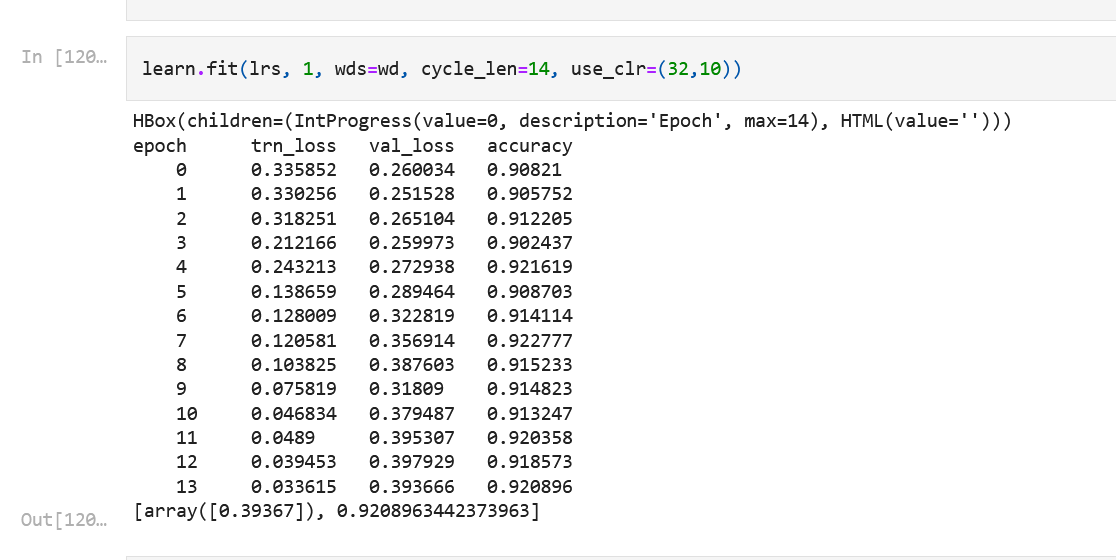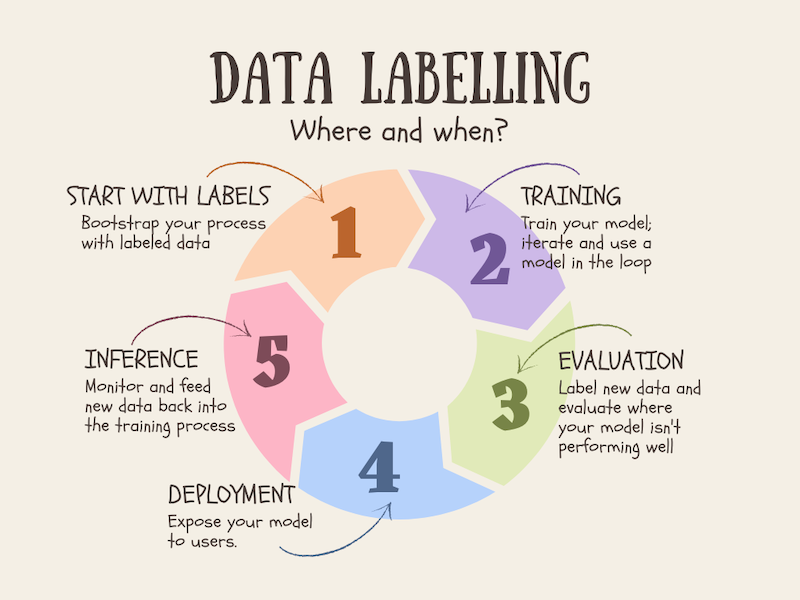## Why trying out simple baseline models?

I have been working on open source project to work on Malayalam text classification approaches. If you want to follow along the previous blogs check the tag #malayalamtextmodels

One of the most under-rated advices which I actually learned from the Approaching almost any Machine learning Problem(AAAMLP) by Abhishek Thakur was to start always with simple models and to create a baseline first. This is something Jeremy also repeatedly emphasises:

So we should always be careful to benchmark simple models, as see if they're good enough for our needs. In practice, you will often find that simple models will have trouble providing adequate accuracy for more complex tasks, such as recommendation systems, NLP, computer vision, or multivariate time series. But there's no need to guess -- it's so easy to try a few different models, there's no reason not to give the simpler ones a go too!

source:7-how-random-forests-really-work

## Doing modeling with simple approaches

Instead of directly trying out transformers models, I first thought of working with some simple models and see how well they perform. Since I had previously read AAAMLP and seen Abhisheks chapter on how trying out simple techniques in IMDB dataset have him impressive results. I also thought of trying the same approaches.

What follows is my attempt to follow steps initially outlined in AAAMLP book.My code doesn't depart from the original code in book much.

Let's start off by importing libraries:

import nltk
import pandas as pd
from sklearn.model_selection import train_test_split
from nltk.tokenize import word_tokenize
from sklearn import linear_model
from sklearn import naive_bayes
from sklearn import metrics
from sklearn import model_selection
from sklearn import preprocessing
from sklearn.feature_extraction.text import CountVectorizer
from sklearn.feature_extraction.text import TfidfVectorizer

nltk.download("punkt")

[nltk_data] Downloading package punkt to
[nltk_data]     /home/kurianbenoy/nltk_data...
[nltk_data]   Package punkt is already up-to-date!

True

For training, I used a privately shared dataset with me which contained news articles with their associated labels. It contained almost 9000+ sentences labelled in 6 categories of news like Sports, Kerala, Business, Gulf, India, Entertainment.

df["labels"].value_counts()

Kerala           3847
Entertainment    1968
Sports           1061
Gulf             1034
India             881
Name: labels, dtype: int64

### Logistic regression + CountVectorizer

One of the first models shared was about using Logistic regression and Count Vectorizer model in AAAMLP book. Let's see how well it performs in our dataset.

count_vec = CountVectorizer(tokenizer=word_tokenize, token_pattern=None)

%%time
count_vec.fit(df.text)

CPU times: user 15.3 s, sys: 196 ms, total: 15.5 s
Wall time: 15.5 s

CountVectorizer(token_pattern=None,
tokenizer=<function word_tokenize at 0x7f940dc34d30>)
In a Jupyter environment, please rerun this cell to show the HTML representation or trust the notebook.
%%time
xtrain = count_vec.transform(df.text)
model = linear_model.LogisticRegression()
model.fit(xtrain, df.labels)

CPU times: user 4min 27s, sys: 7.5 s, total: 4min 35s
Wall time: 1min 2s

/home/kurianbenoy/mambaforge/lib/python3.9/site-packages/sklearn/linear_model/_logistic.py:444: ConvergenceWarning: lbfgs failed to converge (status=1):
STOP: TOTAL NO. of ITERATIONS REACHED LIMIT.

Increase the number of iterations (max_iter) or scale the data as shown in:
https://scikit-learn.org/stable/modules/preprocessing.html
Please also refer to the documentation for alternative solver options:
https://scikit-learn.org/stable/modules/linear_model.html#logistic-regression
n_iter_i = _check_optimize_result(

LogisticRegression()
In a Jupyter environment, please rerun this cell to show the HTML representation or trust the notebook.
data = {
"text": [
"സ്കൂബാഡൈവിങ്ങ്, സ്നോർക്കേലിങ്ങ്, സ്പീഡ്ബോട്ടിങ്ങ്, സർഫിങ്ങ് തുടങ്ങിയ കടൽവിനോദങ്ങൾക്കു പേരുകേട്ട ബാലിയിൽ പോയിട്ടും ഇതൊന്നും പരീക്ഷിച്ചില്ല.ധൈര്യം വരാത്തതുകൊണ്ടാണ്. ഇപ്പോൾ ആലോചിക്കുമ്പോൾ ഒരുകൈ നോക്കാമായിരുന്നെന്നുോന്നുന്നു. സാരമില്ല, ബാക്കിവെച്ച ആഗ്രഹങ്ങളാണല്ലോ മുന്നോട്ടുനീങ്ങാനുള്ള പ്രേരണ. അവസരങ്ങൾ ഇനിയുമുണ്ടാകുമെന്ന് കരുതുന്നു."
],
"labels": ["sport"],
}
data

{'text': ['സ്കൂബാഡൈവിങ്ങ്, സ്നോർക്കേലിങ്ങ്, സ്പീഡ്ബോട്ടിങ്ങ്, സർഫിങ്ങ് തുടങ്ങിയ കടൽവിനോദങ്ങൾക്കു പേരുകേട്ട ബാലിയിൽ പോയിട്ടും ഇതൊന്നും പരീക്ഷിച്ചില്ല.ധൈര്യം വരാത്തതുകൊണ്ടാണ്. ഇപ്പോൾ ആലോചിക്കുമ്പോൾ ഒരുകൈ നോക്കാമായിരുന്നെന്നുോന്നുന്നു. സാരമില്ല, ബാക്കിവെച്ച ആഗ്രഹങ്ങളാണല്ലോ മുന്നോട്ടുനീങ്ങാനുള്ള പ്രേരണ. അവസരങ്ങൾ ഇനിയുമുണ്ടാകുമെന്ന് കരുതുന്നു.'],
'labels': ['sport']}
test_df = pd.DataFrame(data)

text labels
0 സ്കൂബാഡൈവിങ്ങ്, സ്നോർക്കേലിങ്ങ്, സ്പീഡ്ബോട്ടിങ... sport
test_df = df.sample(frac=0.1, random_state=1)
test_df.shape

(936, 2)
xtest = count_vec.transform(test_df.text)

preds = model.predict(xtest)
accuracy = metrics.accuracy_score(test_df.labels, preds)
accuracy

0.9989316239316239

You may be wondering, why I haven't deleted all these code piece even after experimenting. This is something, which recently picked up following fastai v5 course. Instead of always deleting after experimenting, keep your experiments also public. But after experimentation is done, wrap it up as function like the one below so you can reuse it in future experiments as well.

def vectorize_evaluate_loop(train_df, test_df):
count_vec = CountVectorizer(tokenizer=word_tokenize, token_pattern=None)
count_vec.fit(train_df.text)
dependent_train = count_vec.transform(train_df.text)
model = linear_model.LogisticRegression()
model.fit(dependent_train, train_df.labels)
dependent_test = count_vec.transform(test_df.text)
predictions = model.predict(dependent_test)
return metrics.accuracy_score(test_df.labels, predictions)

%%time
vectorize_evaluate_loop(df, test_df)

/home/kurianbenoy/mambaforge/lib/python3.9/site-packages/sklearn/linear_model/_logistic.py:444: ConvergenceWarning: lbfgs failed to converge (status=1):
STOP: TOTAL NO. of ITERATIONS REACHED LIMIT.

Increase the number of iterations (max_iter) or scale the data as shown in:
https://scikit-learn.org/stable/modules/preprocessing.html
Please also refer to the documentation for alternative solver options:
https://scikit-learn.org/stable/modules/linear_model.html#logistic-regression
n_iter_i = _check_optimize_result(

CPU times: user 4min 37s, sys: 6.69 s, total: 4min 43s
Wall time: 1min 17s

0.9989316239316239

The function to create k-folds for calculating validation accuracy across K folds of data. It's very important to create good validation sets.

df["kfold"] = -1
df = df.sample(frac=1).reset_index(drop=True)

df.shape

(9363, 3)
Y_value = df.labels.values
kf = model_selection.StratifiedKFold(n_splits=5)

for fold, (text_, value_) in enumerate(kf.split(X=df, y=Y_value)):
df.loc[value_, "kfold"] = fold

for fold_ in range(5):
train_df = df[df.kfold != fold_].reset_index(drop=True)
test_df = df[df.kfold == fold_].reset_index(drop=True)
print(f"Fold value: {fold_}")
print(f"Accuracy: {vectorize_evaluate_loop(train_df, test_df)}")

Fold value: 0

/home/kurianbenoy/mambaforge/lib/python3.9/site-packages/sklearn/linear_model/_logistic.py:444: ConvergenceWarning: lbfgs failed to converge (status=1):
STOP: TOTAL NO. of ITERATIONS REACHED LIMIT.

Increase the number of iterations (max_iter) or scale the data as shown in:
https://scikit-learn.org/stable/modules/preprocessing.html
Please also refer to the documentation for alternative solver options:
https://scikit-learn.org/stable/modules/linear_model.html#logistic-regression
n_iter_i = _check_optimize_result(

Accuracy: 0.8873465029364656
Fold value: 1

/home/kurianbenoy/mambaforge/lib/python3.9/site-packages/sklearn/linear_model/_logistic.py:444: ConvergenceWarning: lbfgs failed to converge (status=1):
STOP: TOTAL NO. of ITERATIONS REACHED LIMIT.

Increase the number of iterations (max_iter) or scale the data as shown in:
https://scikit-learn.org/stable/modules/preprocessing.html
Please also refer to the documentation for alternative solver options:
https://scikit-learn.org/stable/modules/linear_model.html#logistic-regression
n_iter_i = _check_optimize_result(

Accuracy: 0.8777362520021356
Fold value: 2

/home/kurianbenoy/mambaforge/lib/python3.9/site-packages/sklearn/linear_model/_logistic.py:444: ConvergenceWarning: lbfgs failed to converge (status=1):
STOP: TOTAL NO. of ITERATIONS REACHED LIMIT.

Increase the number of iterations (max_iter) or scale the data as shown in:
https://scikit-learn.org/stable/modules/preprocessing.html
Please also refer to the documentation for alternative solver options:
https://scikit-learn.org/stable/modules/linear_model.html#logistic-regression
n_iter_i = _check_optimize_result(

Accuracy: 0.8916177255739456
Fold value: 3

/home/kurianbenoy/mambaforge/lib/python3.9/site-packages/sklearn/linear_model/_logistic.py:444: ConvergenceWarning: lbfgs failed to converge (status=1):
STOP: TOTAL NO. of ITERATIONS REACHED LIMIT.

Increase the number of iterations (max_iter) or scale the data as shown in:
https://scikit-learn.org/stable/modules/preprocessing.html
Please also refer to the documentation for alternative solver options:
https://scikit-learn.org/stable/modules/linear_model.html#logistic-regression
n_iter_i = _check_optimize_result(

Accuracy: 0.875
Fold value: 4

/home/kurianbenoy/mambaforge/lib/python3.9/site-packages/sklearn/linear_model/_logistic.py:444: ConvergenceWarning: lbfgs failed to converge (status=1):
STOP: TOTAL NO. of ITERATIONS REACHED LIMIT.

Increase the number of iterations (max_iter) or scale the data as shown in:
https://scikit-learn.org/stable/modules/preprocessing.html
Please also refer to the documentation for alternative solver options:
https://scikit-learn.org/stable/modules/linear_model.html#logistic-regression
n_iter_i = _check_optimize_result(

Accuracy: 0.8669871794871795


### Naive Bayes Classifier

A bit faster to complete training, yet only difference is it's having less accuracy compared to previous approach.

def naive_bayes_evaluate_loop(train_df, test_df):
count_vec = CountVectorizer(tokenizer=word_tokenize, token_pattern=None)
count_vec.fit(train_df.text)
dependent_train = count_vec.transform(train_df.text)
# changing different model name for function
model = naive_bayes.MultinomialNB()
model.fit(dependent_train, train_df.labels)
dependent_test = count_vec.transform(test_df.text)
predictions = model.predict(dependent_test)
return metrics.accuracy_score(test_df.labels, predictions)

for fold_ in range(5):
train_df = df[df.kfold != fold_].reset_index(drop=True)
test_df = df[df.kfold == fold_].reset_index(drop=True)
print(f"Fold value: {fold_}")
print(f"Accuracy: {naive_bayes_evaluate_loop(train_df, test_df)}")

Fold value: 0
Accuracy: 0.8441003737319808
Fold value: 1
Accuracy: 0.8227442605445809
Fold value: 2
Accuracy: 0.8296849973304858
Fold value: 3
Accuracy: 0.8269230769230769
Fold value: 4
Accuracy: 0.8183760683760684


### Logistic Regression + Tfidf Vectorizer

def tf_idf_evaluate_loop(train_df, test_df):
# note we are using TfidfVectorizer instead of CountVectorizer
count_vec = TfidfVectorizer(tokenizer=word_tokenize, token_pattern=None)
count_vec.fit(train_df.text)
dependent_train = count_vec.transform(train_df.text)
model = linear_model.LogisticRegression()
model.fit(dependent_train, train_df.labels)
dependent_test = count_vec.transform(test_df.text)
predictions = model.predict(dependent_test)
return metrics.accuracy_score(test_df.labels, predictions)

for fold_ in range(5):
train_df = df[df.kfold != fold_].reset_index(drop=True)
test_df = df[df.kfold == fold_].reset_index(drop=True)
print(f"Fold value: {fold_}")
print(f"Accuracy: {tf_idf_evaluate_loop(train_df, test_df)}")

Fold value: 0

/home/kurianbenoy/mambaforge/lib/python3.9/site-packages/sklearn/linear_model/_logistic.py:444: ConvergenceWarning: lbfgs failed to converge (status=1):
STOP: TOTAL NO. of ITERATIONS REACHED LIMIT.

Increase the number of iterations (max_iter) or scale the data as shown in:
https://scikit-learn.org/stable/modules/preprocessing.html
Please also refer to the documentation for alternative solver options:
https://scikit-learn.org/stable/modules/linear_model.html#logistic-regression
n_iter_i = _check_optimize_result(

Accuracy: 0.8478376935397758
Fold value: 1

/home/kurianbenoy/mambaforge/lib/python3.9/site-packages/sklearn/linear_model/_logistic.py:444: ConvergenceWarning: lbfgs failed to converge (status=1):
STOP: TOTAL NO. of ITERATIONS REACHED LIMIT.

Increase the number of iterations (max_iter) or scale the data as shown in:
https://scikit-learn.org/stable/modules/preprocessing.html
Please also refer to the documentation for alternative solver options:
https://scikit-learn.org/stable/modules/linear_model.html#logistic-regression
n_iter_i = _check_optimize_result(

Accuracy: 0.8344901227976508
Fold value: 2
Accuracy: 0.8489054991991457
Fold value: 3
Accuracy: 0.8290598290598291
Fold value: 4

/home/kurianbenoy/mambaforge/lib/python3.9/site-packages/sklearn/linear_model/_logistic.py:444: ConvergenceWarning: lbfgs failed to converge (status=1):
STOP: TOTAL NO. of ITERATIONS REACHED LIMIT.

Increase the number of iterations (max_iter) or scale the data as shown in:
https://scikit-learn.org/stable/modules/preprocessing.html
Please also refer to the documentation for alternative solver options:
https://scikit-learn.org/stable/modules/linear_model.html#logistic-regression
n_iter_i = _check_optimize_result(

Accuracy: 0.8253205128205128


## Evaluating and looking results

I am genuinely surprised by the following:

1. A simple linear regression on this text classification tasks get's close to 87-89% accuracy when evaluated using K-fold validation approach. We haven't done any complex fine tuning or even label_encoding at the moment. Based on improving with some more tweaks, I am trying a few things here.
2. The state of art model for Text classification in Malayalam claims to have got 92% accuracy based on training only on validation dataset, like we have done in sklearn it seems.Source

What is the difference between Vaaku2Vec and this simple model if it's just less than 3% more accurate?

Yet there is a huge difference between ULMFiT approach which Vaaku2Vec and our baseline model. The Vaaku2Vec model has been trained on base model of Malayalam Wikipedia text, so more text corpus will be present and the model has learned from it. In case of our simple baseline model, it has learned just from 9000+ data points only. Now in case of a new word which is not in this dataset, there is a probability it maybe found in Vaaku2Vec model. Yet it's not always true, because words like Covid will defenitely be not recognized by Vaaku2Vec model also.

## Using simple models for labelling

One of the best things about using simple models to train is also that you can use it for data labelling efforts. To be honest, I am still learning more about data-annotation and labelling. My friend Alex is an expert though here and checkout his awesome blog How to get the most out of data annotation. I really loved this image showing 5 steps of datalabelling.Source and credits: Alex

I see more work on data annotation coming soon as part of this project also. With that have a nice day.